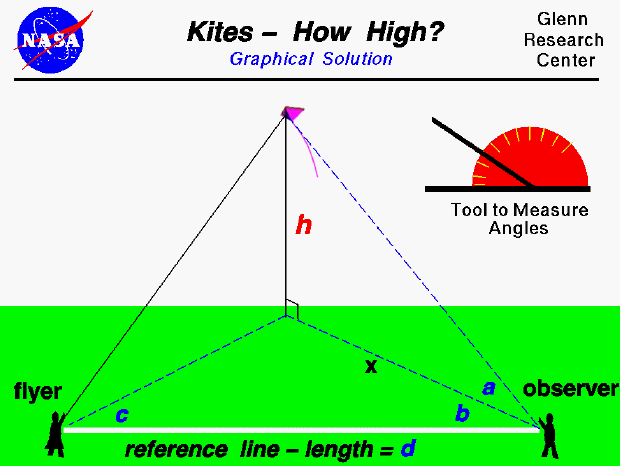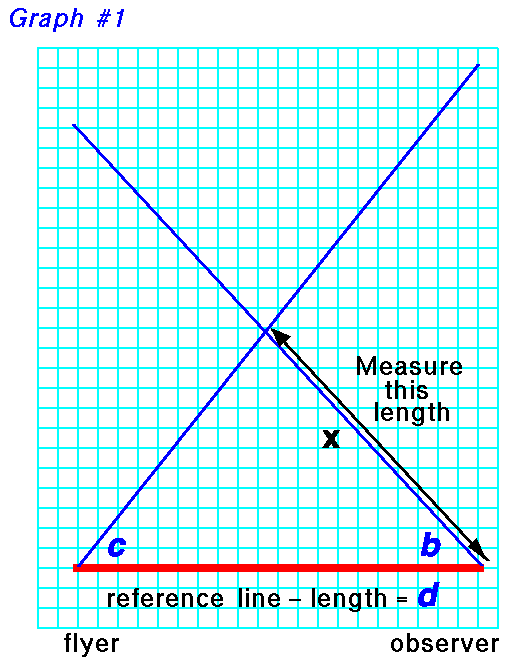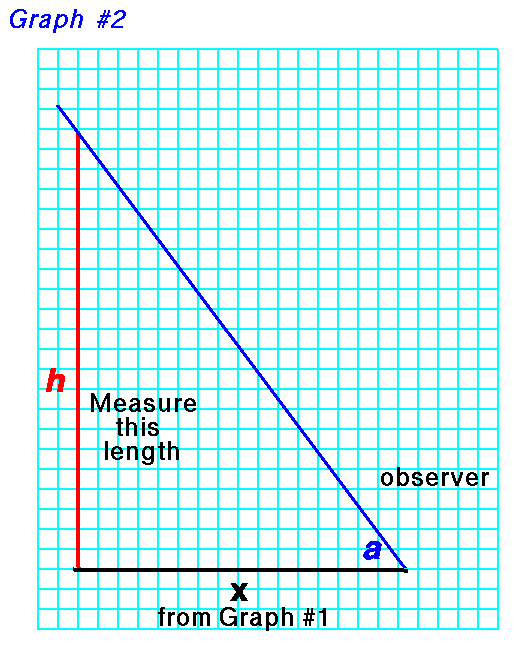An excellent way for students to gain a feel for aerodynamic forces is to fly a kite. Students can also use math techniques learned in school to determine the altitude of the kite during the flight. The same technique can also be applied to a model rocket or to any object flying through the air.

On this page we show a simple way to determine the altitude of a flying kite. The procedure requires an observer in addition to the kite flyer, and a tool (like the one shown in the upper portion of the figure) to measure angles. The observer is stationed some distance (d) from the flyer along a reference line. (You can lay a string of known length along the ground between the flyer and the observer to make this reference line. A long line will produce more accurate results.) To determine the altitude, the flyer calls out "Take Data", and measures the angle (c) between the reference line and the location of the kite. Notice that this measurement is taken parallel to the ground and can be done by the flyer measuring from the kite string to the reference line laid on the ground. When the observer hears the call, "Take Data", the observer must face the kite and measure the angle (a) from the ground to the kite. The observer must then measure the angle (b), parallel to the ground, between the direction the observer is facing and the reference line in the same manner as the flyer.

With the three measured angles and the measured distance between the flyer and the observer, we can use graph paper to build a scale model of the kite in flight and we can determine the altitude (h) of the actual kite. Scale models depend on the mathematical ideas of ratios and proportions which you learn in grade school. To determine the altitude, we first draw the reference line (d) on the graph paper. Make the length of the line on the graph paper some known ratio of the measured length. The length of the line on the graph paper will set the scale of the model. For instance, if the measured length were 100 feet, we might make the line on the graph paper 10 inches long. Then one inch on the graph paper would be equal to 10 feet in the real world. Now draw two lines beginning at the ends of the reference line and inclined at the measured angles (b) and (c).On the graph paper use a ruler to measure the distance (x) from the end of the reference line to the intersection of the two drawn lines.

Now on another piece of graph paper, draw a line of length (x) which you previously measured. At one end of this line, draw another line inclined at the angle (a) which you determined on the actual kite. On the other end of the (x) line, draw a vertical line until it intersects the blue line inclined at angle (a).Now count the blocks (or measure the length) of the vertical line (h). Convert this distance by the scale of the reference line, and you have determined the altitude (h) of the actual kite. For instance, in our example, 1 inch equals 10 feet. If your measured height "h" is 10 inches, the actual kite was flying 100 feet in the air. If you understand the mathematical ideas of trigonometry, you can also calculate the altitude of the kite or model rocket and check your graphical solution.Guided Tours

Beginner's Guide to Aerodynamics
Beginner's Guide to Propulsion
Beginner's Guide to Model Rockets
Beginner's Guide to Kites
Beginner's Guide to Aeronautics